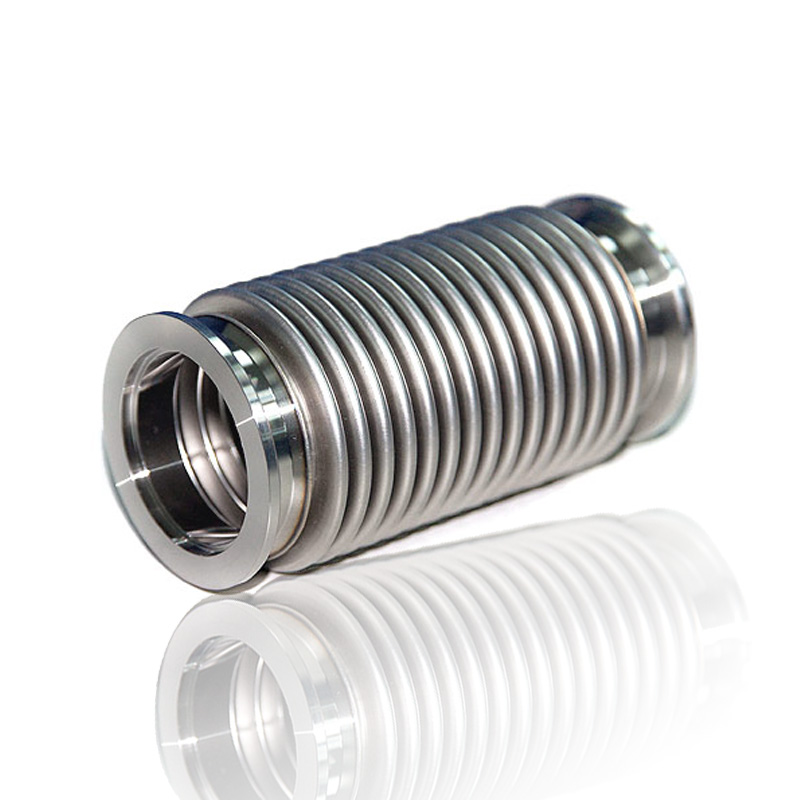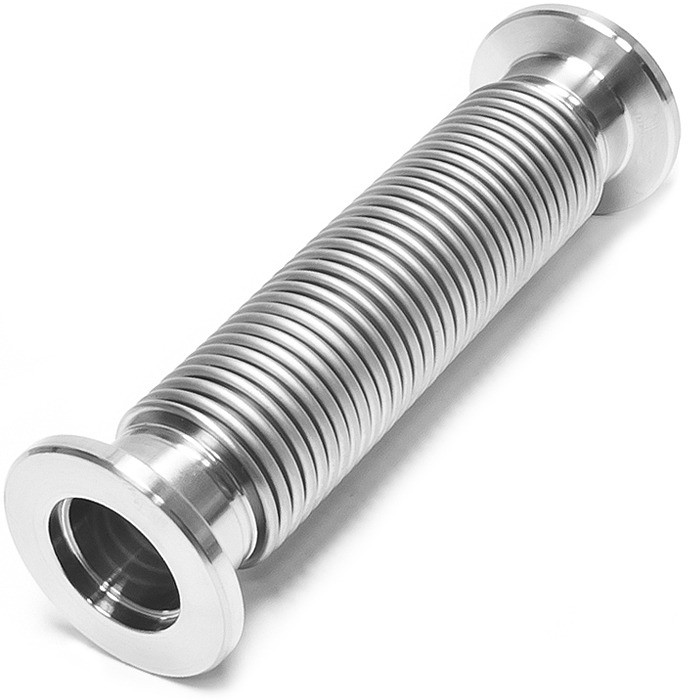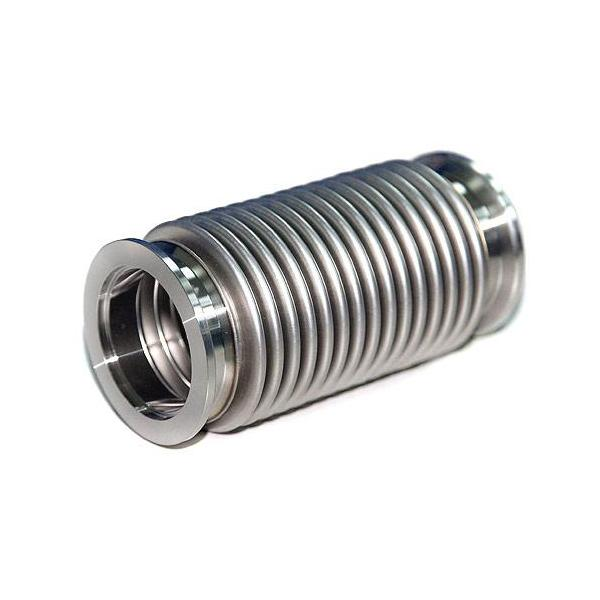﻿ 真空软管接头_成功案例_金属软管接头_不锈钢胶管接头_不锈钢软管接头-众合管业【厂家直销】

400-0393-521

# 真空软管接头1.氦气检测漏缝。

2.零瑕疵

3.耐腐蚀

4.管壁薄

5.波峰波谷均匀

6.焊接工艺优秀DN6=1/8=1分  DN8=1/4=2分  DN10=3/8=3分  DN15=1/2=4分

DN20=3/4=6分  DN25=1寸 DN32=1.2寸=1-1/4  DN40=1.5寸=1-1/2

DN50=2寸  DN65=2.5寸=2-1/2  DN80=3寸 DN100=4寸• 400-0393-521
400-0393-521
•• QQ：1007889475